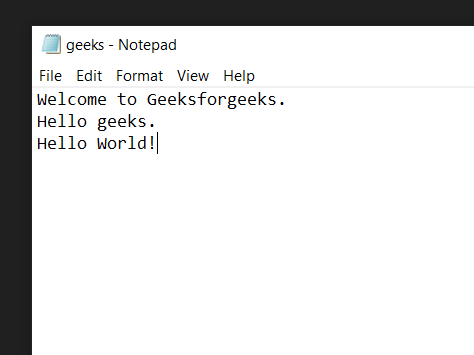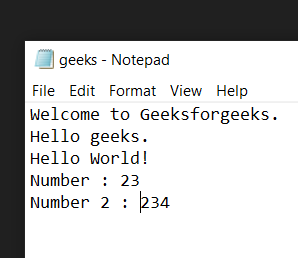# Python Program to Count Words in Text File

In this article, we are going to see how to count words in Text Files using Python.

### Example 1: Count String Words

First, we create a text file of which we want to count the number of words. Let this file be SampleFile.txt with the following contents:

#### File for demonstration:## Python3

 `# creating variable to store the` `# number of words` `number_of_words ``=` `0`   `# Opening our text file in read only` `# mode using the open() function` `with ``open``(r``'SampleFile.txt'``,``'r'``) as ``file``:`   `    ``# Reading the content of the file` `    ``# using the read() function and storing` `    ``# them in a new variable` `    ``data ``=` `file``.read()`   `    ``# Splitting the data into separate lines` `    ``# using the split() function` `    ``lines ``=` `data.split()`   `    ``# Adding the length of the` `    ``# lines in our number_of_words` `    ``# variable` `    ``number_of_words ``+``=` `len``(lines)`     `# Printing total number of words` `print``(number_of_words)`

Output:

`7`

Explanation:

• Creating a new variable to store the total number of words in the text file. And then open the text file in read-only mode using the open() function.
• Read the content of the file using the read() function and storing them in a new variable. And then split the data stored in the data variable into separate lines using the split() function and then storing them in a new variable. And add the length of the lines in our number_of_words variable.

### Example 2: Count the number of words, not Integer

File for demonstration:Below is the implementation:

## Python3

 `# creating variable to store the` `# number of words` `number_of_words ``=` `0`   `# Opening our text file in read only` `# mode using the open() function` `with ``open``(r``'SampleFile.txt'``,``'r'``) as ``file``:`   `    ``# Reading the content of the file` `    ``# using the read() function and storing` `    ``# them in a new variable` `    ``data ``=` `file``.read()`   `    ``# Splitting the data into separate lines` `    ``# using the split() function` `    ``lines ``=` `data.split()`   `    ``# Iterating over every word in` `    ``# lines` `    ``for` `word ``in` `lines:`   `        ``# checking if the word is numeric or not` `        ``if` `not` `word.isnumeric():          `   `            ``# Adding the length of the` `            ``# lines in our number_of_words` `            ``# variable` `            ``number_of_words ``+``=` `1`   `# Printing total number of words` `print``(number_of_words)`

Output:

`11`

Explanation: Create a new variable to store the total number of words in the text file and then open the text file in read-only mode using the open() function. Read the content of the file using the read() function and storing them in a new variable and then split the data stored in the data variable into separate lines using the split() function and then storing them in a new variable, Iterating over every word in lines using the for loop and check if the word is numeric or not using the isnumeric() function then add 1 in our number_of_words variable.

Whether you're preparing for your first job interview or aiming to upskill in this ever-evolving tech landscape, GeeksforGeeks Courses are your key to success. We provide top-quality content at affordable prices, all geared towards accelerating your growth in a time-bound manner. Join the millions we've already empowered, and we're here to do the same for you. Don't miss out - check it out now!